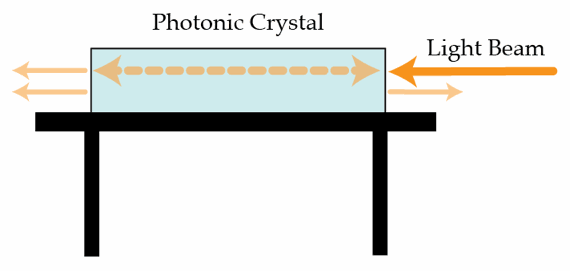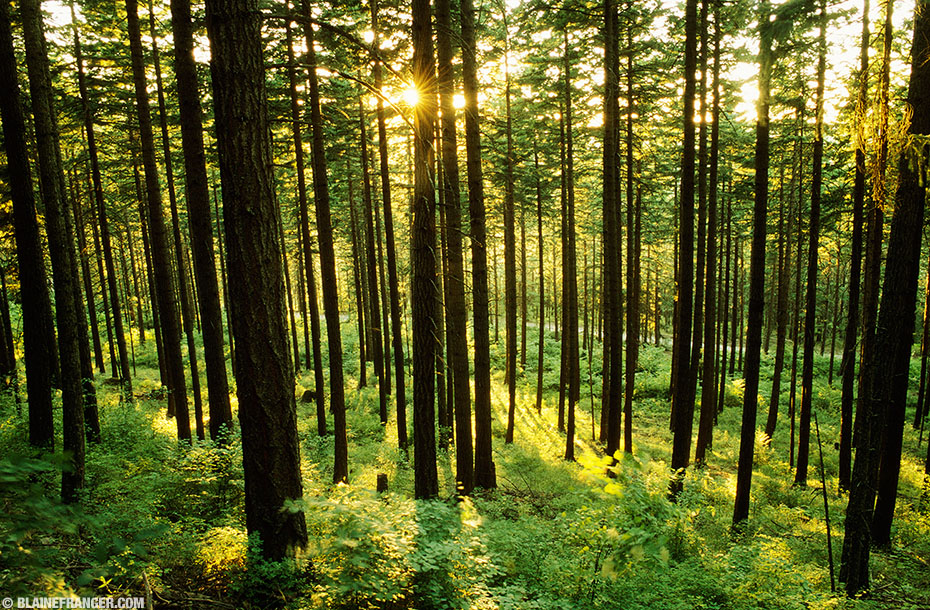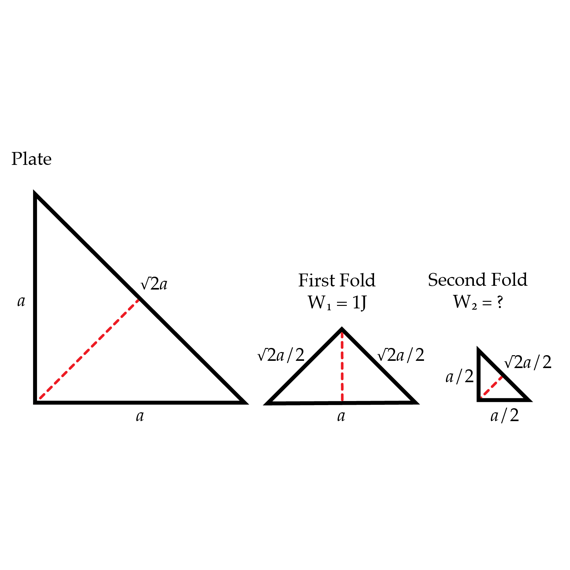Electricity and Magnetism

# E+M Warmups: Level 4 ChallengesPhotonic crystals are materials which have dielectric constant which varies periodically, thus creating many interesting phenomena. One-dimensional photonic crystals are made from parallel layers with varying dielectric constants. We can analyze the unit cell of such crystals by examining the effect a single dielectric crystal has on a beam of light.

Suppose light enters the glass crystal in the image above from the right. Some percentage of the light will exit from the left and some from the right. The transmission coefficient at the air/glass interface is $t = 0.7$, and is the same in both directions. How much of the light in % exits the crystal on the left?

Consider an infinitely long wire made of insulating material which carries a uniform linear charge density $\lambda$. An electron is given a suitable velocity $v$ at a distance of $r$ meters from the wire such that the electron moves in a circular orbit afterwards. Find the minimum value of $r$ for which the above system is stable and the electron does not radiate.

Assumptions and Hints

• Bohr proposed that the angular momentum of an electron is quantized, so that it comes in multiples of $\hbar = h/2\pi$.
• The magnitude of $\lambda$ is equal to the magnitude of $\dfrac{\epsilon_{o}}{200\pi m_{e}e}$
• $m_{e}$ is the mass of an electron.
• $e$ is the charge of an electron.Folk tales are filled with cautionary tales about getting lost in the middle of a dark forest. This trope is not just a convenience for story tellers, it is a lesson about light scattering. As one goes farther into a forest, photons have had more opportunities to hit a tree and bounce backward out of the forest.

Suppose you find yourself $\text{10 m}$ into a dark forest which is illuminated from a (one) side. The canopy is extremely thick so that no light penetrates from above. Moreover, the forest consists of cylindrical trees with average radius $\bar{r}$ which are randomly distributed with number density $\rho$ (i.e. 2 trees per square meter).

What fraction of the incoming light the can you see, on average?

Assumptions

• Ignore second scattering events, if a photon hits a tree once, it exits the forest forever.
• $\bar{r}=6\text{ cm}$
• $\rho = 2\text{ tree m}^{-2}$

The charged particles from solar eruptions hit Earth mainly around the North and South poles (and cause auroras). This is because our Earth is similar to a big magnet. The Earth generates a magnetic field and this magnetic field funnels the charged particles towards the poles. In order to see an example of this funneling, we can think of the following problem:

Consider each magnetic pole separately (so there's only one pole in the problem). The magnetic field near a single pole is $\vec{B} = k\vec{r}/r^3=k\hat{r}/r^2$ where $\vec{r}$ is the radial vector point of the pole and the point of interest and $\hat{r}=\vec{r}/r$ is the radial unit vector. The sign of $k$ is opposite for the North and South poles. If there's an electric charge moving in that magnetic field, its trajectory is on a surface of a cone, i.e. a big funnel. Find the vertex angle (the angle between the axis and a line on the surface of the cone) in degrees with these given initial conditions: the distance between the charge and the pole is $r = 1~\mbox{m}$ and the velocity vector of the charge is $v = 2~\mbox{m/s}$ perpendicular to the line connecting the pole and the charge. We will consider a north pole and so let $k = 3~\mbox{T}\cdot\mbox{m}^2$. The charge of the particle is $q = 4~\mbox{C}$ and the mass is $m = 5~\mbox{kg}$.

Details and assumptions

• Hint: For anyone who has not taken electromagnetism yet, the force on the charge particle is given by the Lorentz force law: $\vec{F}=q\vec{v} \times \vec {B}$.

A thin uniformly charged dielectric plate has the shape of a right isosceles triangle. The plate is folded to obtain a similar triangle. The work required to fold the plate is $W_{1}=1J$. What is the work in Joules $W_{2}$ required to fold it once more as in shown in the figure?×